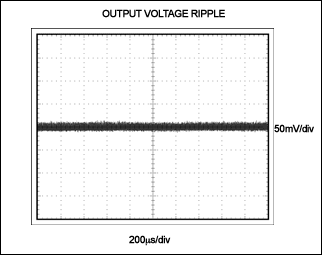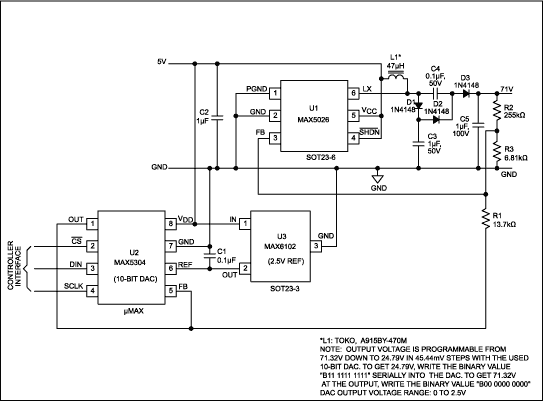# Low-Noise APD Bias Circuit

### Abstract

This circuit generates and controls a low-noise bias voltage for avalanche photodiodes (APDs) used in optical communications. The variable voltage controls the avalanche gain of the APD to optimize sensitivity in a fiber-optic receiver. The circuit employs a low-noise fixed-frequency PWM boost converter with an inductor operating in discontinuous-current mode. Slow switching of the internal MOSFET reduces high-frequency spikes for low-noise performance. A complete circuit is provided, and an extended circuit is suggested. The latter employs an ADC for digital control allowing a microcontroller to read a thermistor to provide temperature compensation by referring to a lookup table.

Avalanche photodiodes (APDs) are used as receiving detectors in optical communications. The APD's high sensitivity and wide bandwidth make it popular with designers. APDs operate with a reverse voltage across the junction that enables the creation of electron-hole pairs in response to incident radiation. The electron-hole pairs are then swept by the applied field and converted to a current that is proportional to the radiation intensity.

Applying a variable reverse-bias voltage across the device junction creates a variable avalanche gain during APD operation. In turn, varying the avalanche gain optimizes sensitivity in the fiber-optic receiver. To achieve satisfactory levels of avalanche gain, however, many APDs require high reverse-bias voltages in the 40V to 60V range, and some require voltages as high as 80V.

A disadvantage of the APD is that avalanche gain depends on temperature and varies with the manufacturing process. Thus, for typical systems in which the APD must operate at constant gain, the high-voltage bias must vary to compensate for the effects of the temperature and manufacturing process on the avalanche gain. To achieve constant gain in a typical APD supply, the temperature coefficient must be maintained at approximately +0.2%/°C, which corresponds to 100mV/°C.

### APD Power Supply

Many methods exist for adjusting the output voltage of a power supply to compensate for temperature-induced gain variations of the APD. APD modules contain temperature-measuring devices such as thermistors, which can be connected directly to the power supply for output-voltage adjustment. In some systems, a microcontroller (µC) reads the resistance value and then issues necessary bias-adjustment commands to the power supply.

The schematic of an APD bias power supply (Figure 1) is based on a low-noise, fixed-frequency PWM boost converter (U1) with an inductor that operates in discontinuous-current mode. Switching times have been intentionally slowed to reduce the high-frequency spikes that are otherwise present in most cases. Slower switching times reduce the high-frequency di/dt and dv/dt rates, which minimize radiated and coupled noise to surrounding circuits through current loops and capacitances between PC board traces or component pins.Operating an inductor in discontinuous-current mode allows the decaying inductor current to naturally turn the diode to off. The MAX5026 switching frequency is 500kHz, and the internal, lateral-DMOS switching device has an absolute maximum rating of 40V. Operating with the external voltage-doubler network formed by C3, C4, D2, and D3, the circuit produces output voltages up to 71V.

Steady-state operation of the doubler circuit functions as follows: C2 transfers charge to C3 during the on time, when L1 is charging and the LX pin is low (internal DMOS conducting). When the internal DMOS switches off, the inductor current forward biases D1 and D3. Thus, the total voltage presented to capacitor C4 is the sum of VC2 and VC3.

The MAX5026 benefits this application with:

• Slow rise and fall times at the internal FET minimize coupled di/dt and dv/dt noise.
• Discontinuous-mode inductor operation naturally commutates D1, virtually eliminating the high di/dt noise caused by reverse recovery in the diode.
• Fixed-frequency, 500kHz PWM operation generates a predictable and easily filtered noise spectrum.
• High integration results in low cost and small size.

With a 5V input, Figure 1's circuit provides more than 1mA of output current at 71V output. Figure 2 shows the range of output-voltage adjustment with respect to the control input voltage, and Figure 3 shows efficiency curves for three output-voltage settings.Set the circuit's output voltage as follows:

VOUT = [VREF × (R2 × R3 + R1 × R2 + R1 × R3) - VC × R2 × R3]/R1 × R3

where VREF = 1.25V and VC is the control input voltage.

Figure 1's circuit has an output ripple of about 100mVP-P at 71V with a 1mA load current. That level can be improved to less than 20mV by placing a low-cost electrolytic capacitor (10µF, 100V Nichicon VX-series) in parallel with a 1µF ceramic capacitor (Figure 4). Additional filtering may be required to reduce the noise to lower levels. The typical noise level for an APD power supply is approximately 2mV. That level is easily achieved with a simple LC filter, given the MAX5026's fixed 500kHz switching frequency.Figure 5's schematic is an APD power supply with digitally adjustable output voltage. A µC in the control loop reads the thermistor value, provides temperature compensation, corrects the thermistor curvature through lookup tables, and compensates for gain variations due to APD manufacturing. In this application circuit, the 10-bit DAC (U2) provides approximately 45mV resolution when varying the output voltage from 25V to 71V.